### 写个 Demo

header('Content-type: image/png');

$image = imagecreatefrompng('card.png');$color = imagecolorallocate($image, 144, 139, 134);$font = getcwd() . '/name.ttf';

$name = "章保滑"; imagettftext($image, 70, 0, 637, 165, $color,$font, $name); imagepng($image);
imagedestroy($image);  同文件夹内要准备好底图和字体，70 是字号，(637, 165) 是文字框的左下角坐标，(144, 139, 134) 是用 QQ 截图工具得到的文字颜色的 RGB 值。在浏览器访问这个 PHP 文件就能得到加上姓名的效果图了：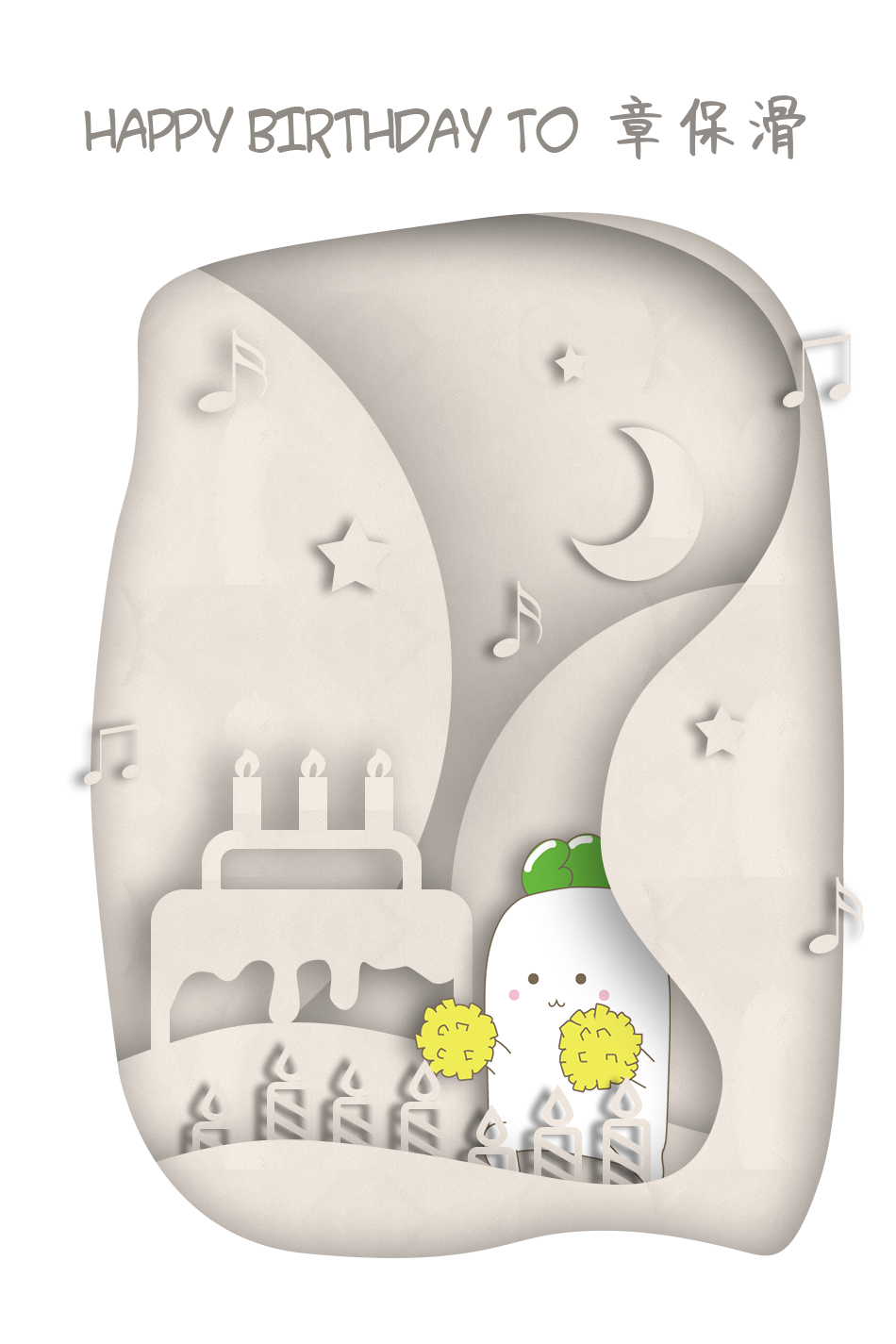哦哟不错哦（主要还是设计小姐姐优秀）。 ### 字体支持问题 ##### 问题简述 接下来解决一下刚刚谈到的艺术字体不支持显示的姓名的问题，因为字体文件这个东西，可以简单认为是给每一个编码的文字都对应设计的一个在计算机上显示的方式，而每个文字是有它自己的唯一编码的，那我们很容易想到如果出现了一个编码的文字，而这个字体并没有设计它如何显示的话，会被怎样处理。再稍微详细一点的相关知识，可以参考 中文字体网页开发指南 高中的时候我时不时会主动帮忙排版一些东西，班上有同学的字是不太常用的那种，然后很多时候为了效果我都会用上特殊字体，所以如果是要用上全班名单的什么文件的话，为了保证整体的统一，可选的字体就那么几种了。当然有时候会先用上艺术字体然后给那个字单独调整字体和大小，让它看起来和旁边的字没有太明显区别就行了。 关于这个字体支持问题，还有个题外话，就是之前有人提议一个字体如果碰到不支持的文字就直接给显示成带叉的方块，这样可以给排版的人一个提醒，因为他发现如果默认使用字体回落的方式的话，会导致带有不常见字的句子同时被多种字体应用，排出来的会是一行千奇百怪高低不平的字，这让他感到非常难受。 在翻数据库的时候我也意外发现了一个 “堃” 字是不被这次用的艺术字体支持的，所以可以用它来做测试样例，尝试实现一个自动字体回落的功能。不过其实它还不是特别不常见，我见过的最不被特殊字体支持的字是 “隺” 字，没错就是我刚刚提到的高中同学的名字里的那个字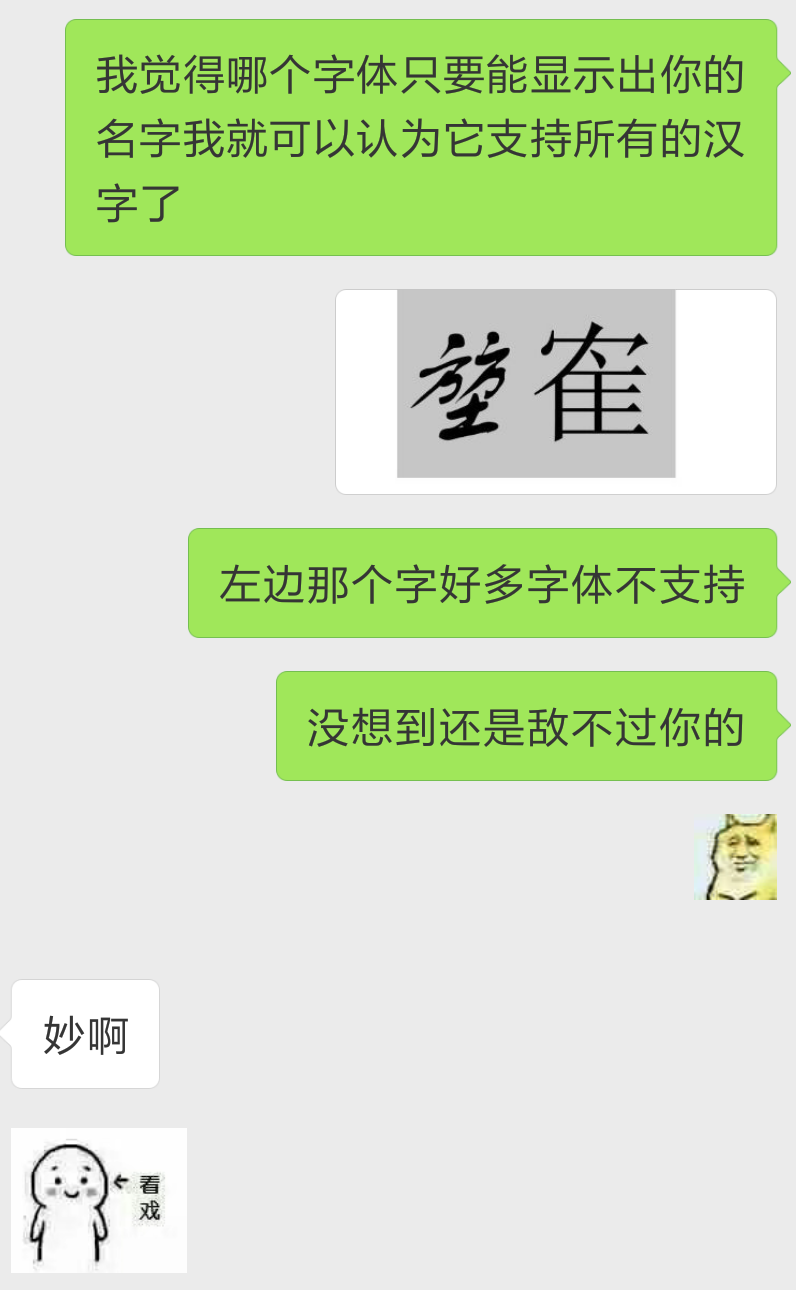在这里感谢这位同学的友情出场（ ##### 解决方案 那我当然是不可能自己想出来的，不过明显这么常见的问题搜一下就知道了。前面说过链接都在各个代码文件的开头所以这里就不给啦，主要的思路是提取出字体文件的相关信息，然后计算一个字符的码位（Code point），再从字体文件的信息里判断出这个码位是不是被它支持。 获取码位的代码是下面这样的，我根据评论区的一个提示稍微修改了一下让它能支持的范围广一点： private function ord_utf8($c) {
$byte0 = ord(substr($c, 0));
if ($byte0 < 0x80) return$byte0;

$byte1 = ord(substr($c, 1));
if ($byte0 < 0xE0) return (($byte0 & 0x1F) << 6) + ($byte1 & 0x3F);$byte2 = ord(substr($c, 2)); return (($byte0 & 0x0F) << 12) + (($byte1 & 0x3F) << 6) + ($byte2 & 0x3F);
}


foreach($font->getData('cmap', 'subtables') as$_subtable) {
if ($_subtable['platformID'] == 3 &&$_subtable['platformSpecificID'] == 1) {
$subtable =$_subtable;
break;
}
}

$ord =$this->ord_utf8($char); if (isset($subtable['glyphIndexArray'][$ord])) return true; return false; }  秉承着作为一个辣鸡的“能用就行”的信念我就没去深究他是怎么做的了，毕竟看了也搞不懂啊入参 $char 是单个的字符，$font 由调用 charInFont 的数组遍历部分传入。剩下的问题就是要把一个姓名分割成其各个单字符组成的数组，这个毕竟是 UTF-8 编码的多字节字符组成的串，不能直接用切割一般字符串的方法，不过很快就找到个操作，再整合一下把字体转为所需的库的 Font 对象的操作以及遍历数组各字符进行判断的逻辑，用于判断整个姓名字符串是否被一个字体文件支持的代码就有了： public function isStringValid($str, $font_path) {$font = Font::load($font_path); if ($font instanceof Collection) {
$font =$font->getFont(0);
}

$chars = preg_split('//u',$str, null, PREG_SPLIT_NO_EMPTY);
foreach ($chars as$char) {
if (!$this->charInFont($char, $font)) return false; } return true; }  这里用到的两个第三方类分别是 Fontlib 库里面的 FontLib\FontFontLib\TrueType\Collection，两个传入参数分别是姓名和字体文件的路径。 ##### 单元测试 继续之前当然是要先测试一下这个东西写得有没有问题，只要 xjb 构造那么十几二十个样例姓名，然后遍历跑一遍就可以了，为了稳一点我们准备个比较通用的字体文件同样跑一遍然后对比一下，结果参考 Sample/CLITest_result.txt，可以看到是完全符合预期的，爽。 ### 字体大小 因为给到用来填名字的就一个框，可是各种名字的长短不同，全部统一的字体大小肯定效果不好。我也没想到什么一劳永逸的解决办法，因为总的情况也不多，就一个个肉眼观察看起来最舒服的大小然后手动记录了 2333： public function getSize($text) {
$fontSize = [ 87, // == 2 characters => '李华' 70, // == 3 characters => '章保滑' 63, // == 4 characters => '章保滑华' 52, // == 5 characters => '恶魔喵喵喵' 35, // > 5 characters => '恶魔喵喵喵喵' 43 // characters with interval => '尼古拉斯·章保滑' ]; return$fontSize[$this->parseIndex($text)];
}


（不要在意注释里奇奇怪怪的示例

public function parseIndex($text) { if (strpos($text, '·') !== false) return 5;    // characters with interval

$len =$this->charLen($text); if ($len < 6) return $len - 2; // [2, 5] characters return 4; // > 5 characters }  如果名字带点的话直接返回最后一个字号，后面处理的时候会把点号换成换行符然后让点号前后的字分两行排。其它的就是直接返回长度了，长度大于 5 的就给个比较小的字号直接往左排起。charLen() 用的是和上面分割姓名为数组同样的实现方法： public function charLen($text) {
return count(preg_split('//u', $text, null, PREG_SPLIT_NO_EMPTY)); }  这两个函数的测试结果同样在 Sample/CLITest_result.txt，稳的。 ### 居中对齐 既然姓名是放在一个框里面的，而各个姓名的字号大小又不一样，如果都是往左开始排的话难免会有一些难看，所以就要想想能不能让姓名在这个给定范围的框里面居中对齐了。 最开始我用的是和字号一样的一个个手动调的方式记录下一个数组然后去分析当前姓名适用哪种情况，但是写起来感觉相当吔。后来发现有个 imagettfbbox 函数，能用和我们写姓名到图片上的 imagettftext 函数一样的参数得到这部分文字的边角坐标值，于是用这个得到的文字宽高值和允许放姓名的外框的值就可以计算出文字相对框边界的位置，让它水平和竖直都居中。 不过这个实现是有点问题的，后面“细节还是很重要”一节会详谈。 ### 绘制参考线 接下来画几条参考线来看看文字是不是确实如我们所想的居中在外框里，并且内容和原有的文字在同一个高度。为了不占太多空间这里就把参数表里面的换行去掉了： // Outer Rectangle imagerectangle($image, TextBox::$RECT['left'], TextBox::$RECT['top'], TextBox::$RECT['right'], TextBox::$RECT['bottom'], $color); // Inner Rectangle imagerectangle($image, $bounding['upper_left_x'],$bounding['upper_left_y'], $bounding['lower_right_x'], bounding['lower_right_y'],$color);

// Text Height Lines
imageline($image, 000,$bounding['upper_left_y'], 950, $bounding['upper_left_y'],$color);
imageline($image, 000,$bounding['lower_right_y'], 950, $bounding['lower_right_y'],$color);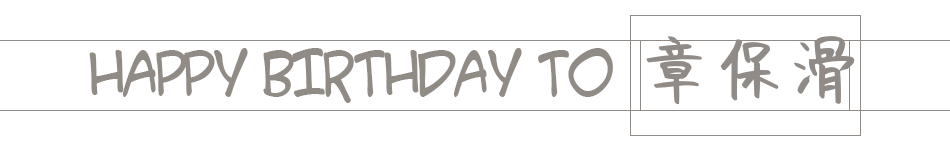### 细节还是很重要

##### 解决问题

print_r(imagettfbbox(70, 0, $font,$name));
// Array (  => 9  => 5  => 218  => 5  => 218  => -65  => 9  => -65 )


$$\begin{matrix} (9, 5) & (218, 5) \\ (9, -65) & (218, -65) \end{matrix}$$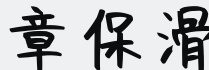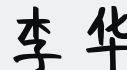##### 再次检验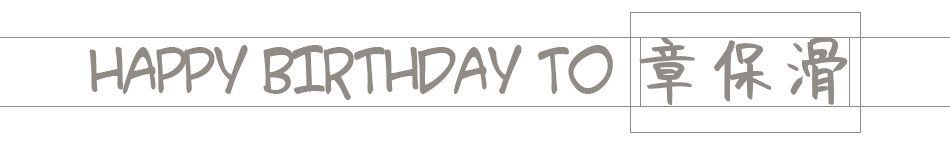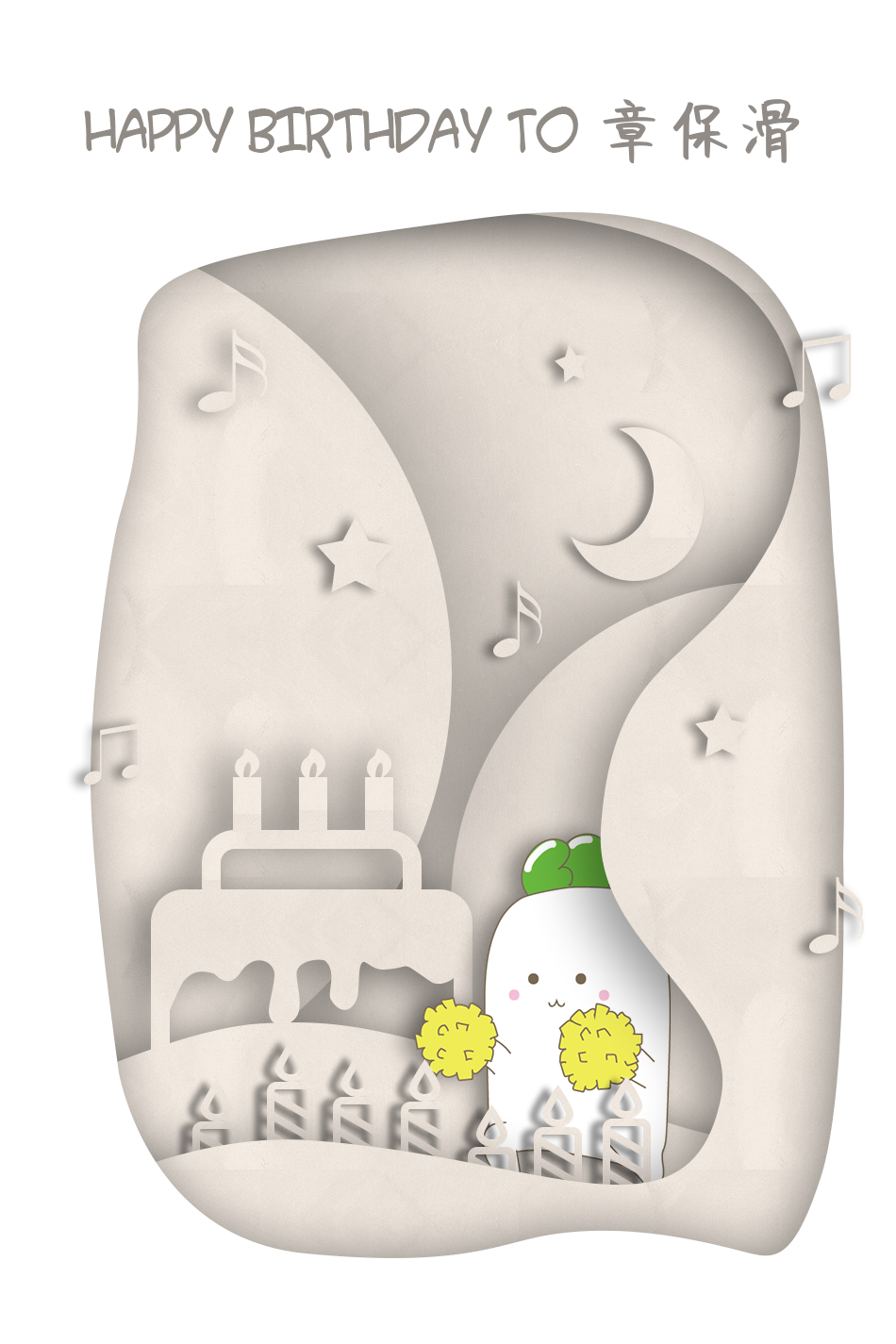### 邮件发送模块

##### 图片导入形式

$this->mailer->addEmbeddedImage($card, 'card');
// Then use cid:card as value of src in HTML code


// HTML code mail body
$this->mailer->Body =$body;
// Plain text as a fallback for clients that does not support HTML render
$this->mailer->AltBody =$altbody;
// Set the default format as HTML first
$this->mailer->isHTML(true);  ##### 另一些细节 考虑一下手机端和电脑端的布局情况，为了避免图片太宽不好看，设置个最大显示宽度让它自己缩放到合适大小，这个时候还需要让图片在水平方向上居中，直接一个 margin: auto 就 OK 了。再稍微调整一下几个细节的地方，加上常规的编码和兼容性设置，最后写出的 HTML 内容也就是 $body 的值是这样的：

<meta charset="UTF-8">
<meta http-equiv="X-UA-Compatible" content="IE=Edge">
<meta name="viewport" content="width=device-width,initial-scale=1.0,maximum-scale=1.0,user-scalable=no">
<div style="margin: auto; max-width: 577px;">
<img style="width: 100%;" src="cid:card">
<img style="width: 100%;" src="cid:line">
<p>生日快乐 (づ￣ ³￣)づ</p>
<p style="text-align: right;">—— 百步梯</p><br>
</div>


### 部署上线

0 11 * * * cd path/to/directory && php index.php
0 10 1 * * cd path/to/directory && php remind.php